Courses

# CAM And Balancing Of Rigid Rotors And Field Balancing - MCQ Test 2

## 25 Questions MCQ Test Mock Test Series - Mechanical Engineering (ME) for GATE 2020 | CAM And Balancing Of Rigid Rotors And Field Balancing - MCQ Test 2

Description
This mock test of CAM And Balancing Of Rigid Rotors And Field Balancing - MCQ Test 2 for Mechanical Engineering helps you for every Mechanical Engineering entrance exam. This contains 25 Multiple Choice Questions for Mechanical Engineering CAM And Balancing Of Rigid Rotors And Field Balancing - MCQ Test 2 (mcq) to study with solutions a complete question bank. The solved questions answers in this CAM And Balancing Of Rigid Rotors And Field Balancing - MCQ Test 2 quiz give you a good mix of easy questions and tough questions. Mechanical Engineering students definitely take this CAM And Balancing Of Rigid Rotors And Field Balancing - MCQ Test 2 exercise for a better result in the exam. You can find other CAM And Balancing Of Rigid Rotors And Field Balancing - MCQ Test 2 extra questions, long questions & short questions for Mechanical Engineering on EduRev as well by searching above.
QUESTION: 1

Solution:
QUESTION: 2

Solution:
QUESTION: 3

### Which one of the following sets of elements are quick acting clamping elements for fixtures?

Solution:
QUESTION: 4

A statically-balanced system is shown in the given Figure. Two equal weights W, each with an eccentricity e, are placed on opposite sides of the axis in the same axial plane. The axial distance between them is 'a'. The total dynamic reactions at the supports will be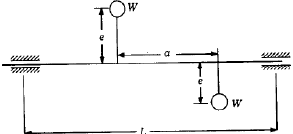Solution: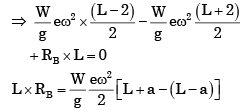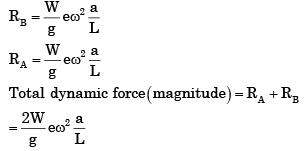QUESTION: 5

The choice of displacement diagram during rise or return of a follower of a cam-follower mechanism is based on dynamic considerations. For high speed cam follower mechanism, the most suitable displacement for the follower is

Solution:
QUESTION: 6

A rotor supported at A and B, carries two masses as shown in the given figure. The rotor is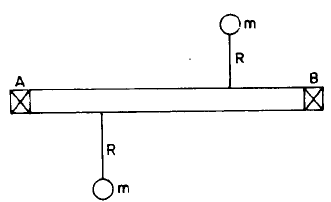Solution:
QUESTION: 7

In a cam drive, it is essential to off-set the axis of a follower to

Solution:
QUESTION: 8

What is the number of nodes in a shaft carrying three rotors?

Solution:
QUESTION: 9

In a cam drive with uniform velocity follower, the slope of the displacement must be as shown in Fig. I. But in actual practice it is as shown in Fig. II (i.e. rounded at the corners).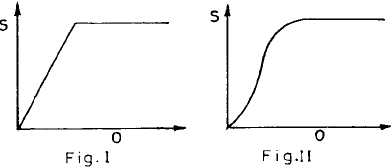This is because of

Solution:
QUESTION: 10

Which one of the following can completely balance several masses revolving in different planes on a shaft?

Solution:
QUESTION: 11

Assertion (A): Cam of a specified contour is preferred to a cam with a specified follower motion.

Reason (R): Cam of a specified contour has superior performance.

Solution:
QUESTION: 12

Masses B1, B2 and 9 kg are attached to a shaft in parallel planes as shown in the figure. If the shaft is rotating at 100 rpm, the mass B2 is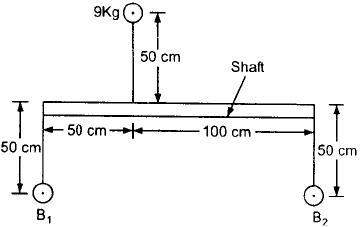Solution:
QUESTION: 13

Which one of the following is an Open Pair?

Solution:

Cam and follower is open pair.

QUESTION: 14

Static balancing is satisfactory for low speed rotors but with increasing speeds, dynamic balancing becomes necessary. This is because, the

Solution:
QUESTION: 15

For a given lift of the follower in a given angular motion of the cam, the acceleration/retardation of the follower will be the least when the profile of the cam during the rise portion is

Solution:
QUESTION: 16

The figures given on right show different schemes suggested to transmit continuous rotary motion from axis A to axis B. Which of these schemes are not dynamically balanced?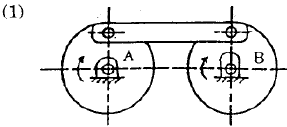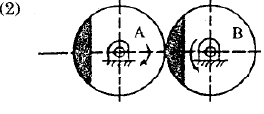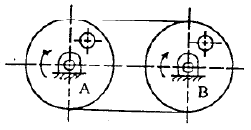Solution:
QUESTION: 17

Consider the following follower motions in respect of a given lift, speed of rotation and angle of stroke of a cam:

1. Cycloidal motion.
2. Simple harmonic motion.
3. Uniform velocity motion.

Which one of the following is the correct sequence of the above in the descending order of maximum velocity?

Solution: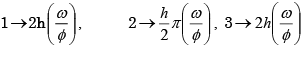QUESTION: 18

Consider the following necessary and sufficient conditions for replacing a rigid body by a dynamical equivalent system of two masses:

1. Total mass must be equal to that of the rigid body.
2. Sum of the squares of radii of gyration of two masses about the c.g. of the rigid body must be equal to square of its radius of gyration about the same point.
3. The c.g. of two masses must coincide with that of the rigid body.
4. The total moment of inertia of two masses about an axis through the c.g. must be equal to that of the rigid body.

Which of the above conditions are correct?

Solution:
QUESTION: 19

What is the maximum acceleration of a cam follower undergoing simple harmonic motion?

Where, h = Stroke of the follower; (ω) = Angular velocity of the cam; ɸ = Cam rotation angle for the maximum follower displacemen

Solution:
QUESTION: 20

Which of the following conditions are to be satisfied by a two-mass system which is dynamically equivalent to a rigid body?

1. The total mass should be equal to that of the rigid body.
2. The centre of gravity should coincide with that of the rigid body.
3. The total moment of inertia about an axis through the centre of gravity must be equal to that of the rigid body.

Select the correct answer using the codes given below:

Solution:

A is false. The centre of gravity of the two masses should coincide with that of the rigid body.

QUESTION: 21

Consider the following statements:

1. For a radial-translating roller follower, parabolic motion of the follower is very suitable for high speed cams.
2. Pitch point on pitch circle of a cam corresponds to the point of maximum pressure angle.

Which of the statements given above is/are correct?

Solution:

For high speed use cycloidal motion.
Pitch point on pitch circle of a cam corresponds to the point of maximum pressure angle.

QUESTION: 22

A rigid rotor consists of a system of two masses located as shown in the given figure. The system is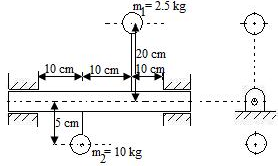Solution:

As centre of masses lie on the axis of rotation.

QUESTION: 23

The shaft-rotor system given above is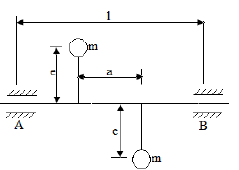Solution:
QUESTION: 24

For the rotor system shown in figure, the mass required for its complete balancing is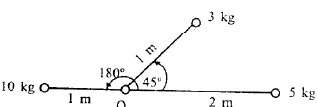Solution: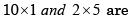balanced each other Unbalance mass is 3 kg at 45°
∴ Balanced system given in figure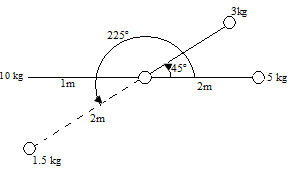QUESTION: 25

Two rotors are mounted on a shaft. If the unbalanced force due to one rotor is equal in magnitude to the unbalanced force due to the other rotor, but positioned exactly 1800 apart, then the system will be balanced

Solution: